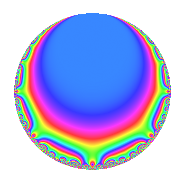# Properties

 Label 8020.2.a.bLevel 8020 Weight 2 Character orbit 8020.a Self dual Yes Analytic conductor 64.040 Analytic rank 0 Dimension 2 CM No Inner twists 1

# Related objects

## Newspace parameters

 Level: $$N$$ = $$8020 = 2^{2} \cdot 5 \cdot 401$$ Weight: $$k$$ = $$2$$ Character orbit: $$[\chi]$$ = 8020.a (trivial)

## Newform invariants

 Self dual: Yes Analytic conductor: $$64.0400224211$$ Analytic rank: $$0$$ Dimension: $$2$$ Coefficient field: $$\Q(\sqrt{3})$$ Coefficient ring: $$\Z[a_1, a_2, a_3]$$ Coefficient ring index: $$1$$ Fricke sign: $$-1$$ Sato-Tate group: $\mathrm{SU}(2)$

## $q$-expansion

Coefficients of the $$q$$-expansion are expressed in terms of $$\beta = \sqrt{3}$$. We also show the integral $$q$$-expansion of the trace form.

 $$f(q)$$ $$=$$ $$q$$ $$+ ( -1 + \beta ) q^{3}$$ $$- q^{5}$$ $$+ 2 \beta q^{7}$$ $$+ ( 1 - 2 \beta ) q^{9}$$ $$+O(q^{10})$$ $$q$$ $$+ ( -1 + \beta ) q^{3}$$ $$- q^{5}$$ $$+ 2 \beta q^{7}$$ $$+ ( 1 - 2 \beta ) q^{9}$$ $$-2 \beta q^{11}$$ $$+ ( -5 + \beta ) q^{13}$$ $$+ ( 1 - \beta ) q^{15}$$ $$+ ( 1 - \beta ) q^{17}$$ $$-4 \beta q^{19}$$ $$+ ( 6 - 2 \beta ) q^{21}$$ $$+ ( 3 - 3 \beta ) q^{23}$$ $$+ q^{25}$$ $$-4 q^{27}$$ $$+ ( 2 - 2 \beta ) q^{29}$$ $$+ ( -2 + 2 \beta ) q^{31}$$ $$+ ( -6 + 2 \beta ) q^{33}$$ $$-2 \beta q^{35}$$ $$+ ( 5 + 3 \beta ) q^{37}$$ $$+ ( 8 - 6 \beta ) q^{39}$$ $$+ ( -2 + 6 \beta ) q^{41}$$ $$-2 q^{43}$$ $$+ ( -1 + 2 \beta ) q^{45}$$ $$+ ( -2 + 4 \beta ) q^{47}$$ $$+ 5 q^{49}$$ $$+ ( -4 + 2 \beta ) q^{51}$$ $$+ ( 7 - 3 \beta ) q^{53}$$ $$+ 2 \beta q^{55}$$ $$+ ( -12 + 4 \beta ) q^{57}$$ $$-8 q^{59}$$ $$+ ( 2 - 4 \beta ) q^{61}$$ $$+ ( -12 + 2 \beta ) q^{63}$$ $$+ ( 5 - \beta ) q^{65}$$ $$+ ( -3 + 3 \beta ) q^{67}$$ $$+ ( -12 + 6 \beta ) q^{69}$$ $$+ ( 6 + 2 \beta ) q^{71}$$ $$+ ( 6 + 4 \beta ) q^{73}$$ $$+ ( -1 + \beta ) q^{75}$$ $$-12 q^{77}$$ $$+ ( 1 + 2 \beta ) q^{81}$$ $$+ ( -2 + 8 \beta ) q^{83}$$ $$+ ( -1 + \beta ) q^{85}$$ $$+ ( -8 + 4 \beta ) q^{87}$$ $$+ ( -2 + 8 \beta ) q^{89}$$ $$+ ( 6 - 10 \beta ) q^{91}$$ $$+ ( 8 - 4 \beta ) q^{93}$$ $$+ 4 \beta q^{95}$$ $$+ ( 9 - \beta ) q^{97}$$ $$+ ( 12 - 2 \beta ) q^{99}$$ $$+O(q^{100})$$ $$\operatorname{Tr}(f)(q)$$ $$=$$ $$2q$$ $$\mathstrut -\mathstrut 2q^{3}$$ $$\mathstrut -\mathstrut 2q^{5}$$ $$\mathstrut +\mathstrut 2q^{9}$$ $$\mathstrut +\mathstrut O(q^{10})$$ $$2q$$ $$\mathstrut -\mathstrut 2q^{3}$$ $$\mathstrut -\mathstrut 2q^{5}$$ $$\mathstrut +\mathstrut 2q^{9}$$ $$\mathstrut -\mathstrut 10q^{13}$$ $$\mathstrut +\mathstrut 2q^{15}$$ $$\mathstrut +\mathstrut 2q^{17}$$ $$\mathstrut +\mathstrut 12q^{21}$$ $$\mathstrut +\mathstrut 6q^{23}$$ $$\mathstrut +\mathstrut 2q^{25}$$ $$\mathstrut -\mathstrut 8q^{27}$$ $$\mathstrut +\mathstrut 4q^{29}$$ $$\mathstrut -\mathstrut 4q^{31}$$ $$\mathstrut -\mathstrut 12q^{33}$$ $$\mathstrut +\mathstrut 10q^{37}$$ $$\mathstrut +\mathstrut 16q^{39}$$ $$\mathstrut -\mathstrut 4q^{41}$$ $$\mathstrut -\mathstrut 4q^{43}$$ $$\mathstrut -\mathstrut 2q^{45}$$ $$\mathstrut -\mathstrut 4q^{47}$$ $$\mathstrut +\mathstrut 10q^{49}$$ $$\mathstrut -\mathstrut 8q^{51}$$ $$\mathstrut +\mathstrut 14q^{53}$$ $$\mathstrut -\mathstrut 24q^{57}$$ $$\mathstrut -\mathstrut 16q^{59}$$ $$\mathstrut +\mathstrut 4q^{61}$$ $$\mathstrut -\mathstrut 24q^{63}$$ $$\mathstrut +\mathstrut 10q^{65}$$ $$\mathstrut -\mathstrut 6q^{67}$$ $$\mathstrut -\mathstrut 24q^{69}$$ $$\mathstrut +\mathstrut 12q^{71}$$ $$\mathstrut +\mathstrut 12q^{73}$$ $$\mathstrut -\mathstrut 2q^{75}$$ $$\mathstrut -\mathstrut 24q^{77}$$ $$\mathstrut +\mathstrut 2q^{81}$$ $$\mathstrut -\mathstrut 4q^{83}$$ $$\mathstrut -\mathstrut 2q^{85}$$ $$\mathstrut -\mathstrut 16q^{87}$$ $$\mathstrut -\mathstrut 4q^{89}$$ $$\mathstrut +\mathstrut 12q^{91}$$ $$\mathstrut +\mathstrut 16q^{93}$$ $$\mathstrut +\mathstrut 18q^{97}$$ $$\mathstrut +\mathstrut 24q^{99}$$ $$\mathstrut +\mathstrut O(q^{100})$$

## Embeddings

For each embedding $$\iota_m$$ of the coefficient field, the values $$\iota_m(a_n)$$ are shown below.

For more information on an embedded modular form you can click on its label.

Label $$\iota_m(\nu)$$ $$a_{2}$$ $$a_{3}$$ $$a_{4}$$ $$a_{5}$$ $$a_{6}$$ $$a_{7}$$ $$a_{8}$$ $$a_{9}$$ $$a_{10}$$
1.1
 −1.73205 1.73205
0 −2.73205 0 −1.00000 0 −3.46410 0 4.46410 0
1.2 0 0.732051 0 −1.00000 0 3.46410 0 −2.46410 0
 $$n$$: e.g. 2-40 or 990-1000 Significant digits: Format: Complex embeddings Normalized embeddings Satake parameters Satake angles

## Inner twists

This newform does not admit any (nontrivial) inner twists.

## Atkin-Lehner signs

$$p$$ Sign
$$2$$ $$-1$$
$$5$$ $$1$$
$$401$$ $$1$$

## Hecke kernels

This newform can be constructed as the kernel of the linear operator $$T_{3}^{2}$$ $$\mathstrut +\mathstrut 2 T_{3}$$ $$\mathstrut -\mathstrut 2$$ acting on $$S_{2}^{\mathrm{new}}(\Gamma_0(8020))$$.